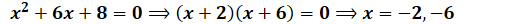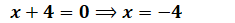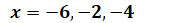It looks like you're using Internet Explorer 11 or older. This website works best with modern browsers such as the latest versions of Chrome, Firefox, Safari, and Edge. If you continue with this browser, you may see unexpected results.

# Math

Some of the content of this guide was modeled after a guide originally created by the Openstax and has been adapted for the GPRC Learning Commons in September 2020. This work is licensed under a Creative Commons BY 4.0 International License.Dividing Rational Expressions:

##### Step 2) Multiply the first fraction by the reciprocal of the second fraction.##### Step 3) Multiply the numerators with each other and the denominators with each other##### Step 4) Simplify the result..

##### Step 1) We factor the numerator and the denominator in the first fraction.##### therefore, we have##### The original division becomes:##### Step 2) Now, we convert division to a multiplication:##### Step 3) We multiply the numerators together and the denominators together:##### Step 4) We simplify the result..

##### To find the non-permissible values, one has to set the denominator of the first fraction and second fraction as well as the numerator of the second fraction equal to zero and solve for the variable:Now, we set the denominator of the second fraction equal to zero and solve for x:#####ATTENTION!! In order to find the non-permissible values of a division, one has to set the denominator of the first fraction and second fraction as well as the numerator of the second fraction equal to zero and solve for the variable.